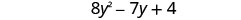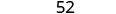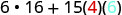Skip to main content
$$\newcommand{\id}{\mathrm{id}}$$ $$\newcommand{\Span}{\mathrm{span}}$$ $$\newcommand{\kernel}{\mathrm{null}\,}$$ $$\newcommand{\range}{\mathrm{range}\,}$$ $$\newcommand{\RealPart}{\mathrm{Re}}$$ $$\newcommand{\ImaginaryPart}{\mathrm{Im}}$$ $$\newcommand{\Argument}{\mathrm{Arg}}$$ $$\newcommand{\norm}{\| #1 \|}$$ $$\newcommand{\inner}{\langle #1, #2 \rangle}$$ $$\newcommand{\Span}{\mathrm{span}}$$

# 6.1: Add and Subtract Polynomials

$$\newcommand{\vecs}{\overset { \rightharpoonup} {\mathbf{#1}} }$$ $$\newcommand{\vecd}{\overset{-\!-\!\rightharpoonup}{\vphantom{a}\smash {#1}}}$$$$\newcommand{\id}{\mathrm{id}}$$ $$\newcommand{\Span}{\mathrm{span}}$$ $$\newcommand{\kernel}{\mathrm{null}\,}$$ $$\newcommand{\range}{\mathrm{range}\,}$$ $$\newcommand{\RealPart}{\mathrm{Re}}$$ $$\newcommand{\ImaginaryPart}{\mathrm{Im}}$$ $$\newcommand{\Argument}{\mathrm{Arg}}$$ $$\newcommand{\norm}{\| #1 \|}$$ $$\newcommand{\inner}{\langle #1, #2 \rangle}$$ $$\newcommand{\Span}{\mathrm{span}}$$ $$\newcommand{\id}{\mathrm{id}}$$ $$\newcommand{\Span}{\mathrm{span}}$$ $$\newcommand{\kernel}{\mathrm{null}\,}$$ $$\newcommand{\range}{\mathrm{range}\,}$$ $$\newcommand{\RealPart}{\mathrm{Re}}$$ $$\newcommand{\ImaginaryPart}{\mathrm{Im}}$$ $$\newcommand{\Argument}{\mathrm{Arg}}$$ $$\newcommand{\norm}{\| #1 \|}$$ $$\newcommand{\inner}{\langle #1, #2 \rangle}$$ $$\newcommand{\Span}{\mathrm{span}}$$

Learning Objectives

By the end of this section, you will be able to:

• Identify polynomials, monomials, binomials, and trinomials
• Determine the degree of polynomials
• Add and subtract monomials
• Add and subtract polynomials
• Evaluate a polynomial for a given value

Quiz

Before you get started, take this readiness quiz.

1. Simplify: $$8x+3x$$.
If you missed this problem, review Exercise 1.3.37.
2. Subtract: $$(5n+8)−(2n−1)$$.
If you missed this problem, review Exercise 1.10.52.
3. Write in expanded form: $$a^{5}$$.
If you missed this problem, review Exercise 1.3.7.

## Identify Polynomials, Monomials, Binomials and Trinomials

You have learned that a term is a constant or the product of a constant and one or more variables. When it is of the form $$ax^{m}$$, where $$a$$ is a constant and $$m$$ is a whole number, it is called a monomial. Some examples of monomial are $$8,−2x^{2},4y^{3}$$, and $$11z^{7}$$.

Definition: Monomials

A monomial is a term of the form $$ax^{m}$$, where $$a$$ is a constant and $$m$$ is a positive whole number.

A monomial, or two or more monomials combined by addition or subtraction, is a polynomial. Some polynomials have special names, based on the number of terms. A monomial is a polynomial with exactly one term. A binomial has exactly two terms, and a trinomial has exactly three terms. There are no special names for polynomials with more than three terms.

Definitions: Polynomials

• polynomial—A monomial, or two or more monomials combined by addition or subtraction, is a polynomial.
• monomial—A polynomial with exactly one term is called a monomial.
• binomial—A polynomial with exactly two terms is called a binomial.
• trinomial—A polynomial with exactly three terms is called a trinomial.

Here are some examples of polynomials.

$\begin{array}{lllll}{\text { Polynomial }} & {b+1} &{4 y^{2}-7 y+2} & {4 x^{4}+x^{3}+8 x^{2}-9 x+1} \\ {\text { Monomial }} & {14} & {8 y^{2}} & {-9 x^{3} y^{5}} & {-13}\\ {\text { Binomial }} & {a+7}&{4 b-5} & {y^{2}-16}& {3 x^{3}-9 x^{2}} \\ {\text { Trinomial }} & {x^{2}-7 x+12} & {9 y^{2}+2 y-8} & {6 m^{4}-m^{3}+8 m}&{z^{4}+3 z^{2}-1} \end{array} \nonumber$

Notice that every monomial, binomial, and trinomial is also a polynomial. They are just special members of the “family” of polynomials and so they have special names. We use the words monomial, binomial, and trinomial when referring to these special polynomials and just call all the rest polynomials.

Example $$\PageIndex{1}$$

Determine whether each polynomial is a monomial, binomial, trinomial, or other polynomial.

1. $$4y^{2}−8y−6$$
2. $$−5a^{4}b^{2}$$
3. $$2x^{5}−5x^{3}−3x + 4$$
4. $$13−5m^{3}$$
5. q
Answer

$$\begin{array}{lll}&{\text { Polynomial }} & {\text { Number of terms }} & {\text { Type }} \\ {\text { (a) }} & {4 y^{2}-8 y-6} & {3} & {\text { Trinomial }} \\ {\text { (b) }} & {-5 a^{4} b^{2}} & {1} & {\text { Monomial }} \\ {\text { (c) }} & {2 x^{5}-5 x^{3}-9 x^{2}+3 x+4} & {5} & {\text { Ponomial }} \\ {\text { (d) }} & {13-5 m^{3}} & {2} & {\text { Binomial }} \\ {\text { (e) }} & {q} & {1} & {\text { Monomial }}\end{array}$$

Example $$\PageIndex{2}$$

Determine whether each polynomial is a monomial, binomial, trinomial, or other polynomial:

1. 5b
2. $$8 y^{3}-7 y^{2}-y-3$$
3. $$-3 x^{2}-5 x+9$$
4. $$81-4 a^{2}$$
5. $$-5 x^{6}$$
Answer
1. monomial
2. polynomial
3. trinomial
4. binomial
5. monomial

Example $$\PageIndex{3}$$

Determine whether each polynomial is a monomial, binomial, trinomial, or other polynomial:

1. $$27 z^{3}-8$$
2. $$12 m^{3}-5 m^{2}-2 m$$
3. $$\frac{5}{6}$$
4. $$8 x^{4}-7 x^{2}-6 x-5$$
5. $$-n^{4}$$
Answer
1. binomial
2. trinomial
3. monomial
4. polynomial
5. monomial

## Determine the Degree of Polynomials

The degree of a polynomial and the degree of its terms are determined by the exponents of the variable. A monomial that has no variable, just a constant, is a special case. The degree of a constant is 0, i.e., it has no variable.

Definition: Degree of a Polynomial

• The degree of a term is the sum of the exponents of its variables.
• The degree of a constant is 0.
• The degree of a polynomial is the highest degree of all its terms.

Let’s see how this works by looking at several polynomials. We’ll take it step by step, starting with monomials, and then progressing to polynomials with more terms.A polynomial is in standard form when the terms of a polynomial are written in descending order of degrees. Get in the habit of writing the term with the highest degree first.

Example $$\PageIndex{4}$$

Find the degree of the following polynomials.

1. 10y
2. $$4 x^{3}-7 x+5$$
3. −15
4. $$-8 b^{2}+9 b-2$$
5. $$8 x y^{2}+2 y$$
Answer
1. $$\begin{array}{ll} & 10y\\ \text{The exponent of y is one. } y=y^1 & \text{The degree is 1.}\end{array}$$
2. $$\begin{array}{ll} & 4 x^{3}-7 x+5\\ \text{The highest degree of all the terms is 3.} & \text{The degree is 3.}\end{array}$$
3. $$\begin{array}{ll} & -15\\ \text{The degree of a constant is 0.} & \text{The degree is 0.}\end{array}$$
4. $$\begin{array}{ll} & -8 b^{2}+9 b-2\\ \text{The highest degree of all the terms is 2.} & \text{The degree is 2.}\end{array}$$
5. $$\begin{array}{ll} & 8 x y^{2}+2 y\\ \text{The highest degree of all the terms is 3.} & \text{The degree is 3.}\end{array}$$

Example $$\PageIndex{5}$$

Find the degree of the following polynomials:

1. −15b
2. $$10 z^{4}+4 z^{2}-5$$
3. $$12 c^{5} d^{4}+9 c^{3} d^{9}-7$$
4. $$3 x^{2} y-4 x$$
5. −9
Answer
1. 1
2. 4
3. 12
4. 3
5. 0

Example $$\PageIndex{6}$$

Find the degree of the following polynomials:

1. 52
2. $$a^{4} b-17 a^{4}$$
3. $$5 x+6 y+2 z$$
4. $$3 x^{2}-5 x+7$$
5. $$-a^{3}$$
Answer
1. 0
2. 5
3. 1
4. 2
5. 3

## Add and Subtract Monomials

You have learned how to simplify expressions by combining like terms. Remember, like terms must have the same variables with the same exponent. Since monomials are terms, adding and subtracting monomials is the same as combining like terms. If the monomials are like terms, we just combine them by adding or subtracting the coefficient.

Example $$\PageIndex{7}$$

Add:$$25 y^{2}+15 y^{2}$$

Answer

$$\begin{array}{ll} & 25 y^{2}+15 y^{2}\\ \text{Combine like terms.} & 40y^{2}\end{array}$$

Example $$\PageIndex{8}$$

Add: $$12 q^{2}+9 q^{2}$$

Answer

21$$q^{2}$$

Example $$\PageIndex{9}$$

Add:$$-15 c^{2}+8 c^{2}$$

Answer

$$-7 c^{2}$$

Example $$\PageIndex{10}$$

Subtract: 16p−(−7p)

Answer

$$\begin{array}{ll} & 16p−(−7p) \\ \text{Combine like terms.} & 23p\end{array}$$

Example $$\PageIndex{11}$$

Subtract: 8m−(−5m).

Answer

13m

Example $$\PageIndex{12}$$

Subtract: $$-15 z^{3}-\left(-5 z^{3}\right)$$

Answer

$$-10 z^{3}$$

Remember that like terms must have the same variables with the same exponents.

Example $$\PageIndex{13}$$

Simplify: $$c^{2}+7 d^{2}-6 c^{2}$$

Answer

$$\begin{array}{ll} & c^{2}+7 d^{2}-6 c^{2} \\ \text{Combine like terms.} & -5 c^{2}+7 d^{2} \end{array}$$

Example $$\PageIndex{14}$$

Add: $$8 y^{2}+3 z^{2}-3 y^{2}$$

Answer

$$5 y^{2}+3 z^{2}$$

Example $$\PageIndex{15}$$

Add: $$3 m^{2}+n^{2}-7 m^{2}$$

Answer

$$-4 m^{2}+n^{2}$$

Example $$\PageIndex{16}$$

Simplify: $$u^{2} v+5 u^{2}-3 v^{2}$$

Answer

$$\begin{array}{ll} &u^{2} v+5 u^{2}-3 v^{2} \\ \text{There are no like terms to combine.} & u^{2} v+5 u^{2}-3 v^{2} \end{array}$$

Example $$\PageIndex{17}$$

Simplify: $$m^{2} n^{2}-8 m^{2}+4 n^{2}$$

Answer

There are no like terms to combine.

Example $$\PageIndex{18}$$

Simplify: $$p q^{2}-6 p-5 q^{2}$$

Answer

There are no like terms to combine.

## Add and Subtract Polynomials

We can think of adding and subtracting polynomials as just adding and subtracting a series of monomials. Look for the like terms—those with the same variables and the same exponent. The Commutative Property allows us to rearrange the terms to put like terms together.

Example $$\PageIndex{19}$$

Find the sum: $$\left(5 y^{2}-3 y+15\right)+\left(3 y^{2}-4 y-11\right)$$

Answer
 Identify like terms.Rearrange to get the like terms together.Combine like terms.Example $$\PageIndex{20}$$

Find the sum: $$\left(7 x^{2}-4 x+5\right)+\left(x^{2}-7 x+3\right)$$

Answer

$$8 x^{2}-11 x+1$$

Example $$\PageIndex{21}$$

Find the sum:$$\left(14 y^{2}+6 y-4\right)+\left(3 y^{2}+8 y+5\right)$$

Answer

$$17 y^{2}+14 y+1$$

Example $$\PageIndex{22}$$

Find the difference: $$\left(9 w^{2}-7 w+5\right)-\left(2 w^{2}-4\right)$$

AnswerDistribute and identify like terms.Rearrange the terms.Combine like terms.Example $$\PageIndex{23}$$

Find the difference: $$\left(8 x^{2}+3 x-19\right)-\left(7 x^{2}-14\right)$$

Answer

$$15 x^{2}+3 x-5$$

Example $$\PageIndex{24}$$

Find the difference: $$\left(9 b^{2}-5 b-4\right)-\left(3 b^{2}-5 b-7\right)$$

Answer

$$6 b^{2}+3$$

Example $$\PageIndex{25}$$

Subtract: $$\left(c^{2}-4 c+7\right)$$ from $$\left(7 c^{2}-5 c+3\right)$$

AnswerDistribute and identify like terms.Rearrange the terms.Combine like terms.Example $$\PageIndex{26}$$

Subtract: $$\left(5 z^{2}-6 z-2\right)$$ from $$\left(7 z^{2}+6 z-4\right)$$

Answer

$$2 z^{2}+12 z-2$$

Example $$\PageIndex{27}$$

Subtract: $$\left(x^{2}-5 x-8\right)$$ from $$\left(6 x^{2}+9 x-1\right)$$

Answer

$$5 x^{2}+14 x+7$$

Example $$\PageIndex{28}$$

Find the sum: $$\left(u^{2}-6 u v+5 v^{2}\right)+\left(3 u^{2}+2 u v\right)$$

Answer

$$\begin{array} {ll} & {\left(u^{2}-6 u v+5 v^{2}\right)+\left(3 u^{2}+2 u v\right)} \\\text{Distribute.} & {u^{2}-6 u v+5 v^{2}+3 u^{2}+2 u v} \\ \text{Rearrange the terms, to put like terms together} & {u^{2}+3 u^{2}-6 u v+2 u v+5 v^{2}} \\ \text{Combine like terms.} & {4 u^{2}-4 u v+5 v^{2}}\end{array}$$

Example $$\PageIndex{29}$$

Find the sum: $$\left(3 x^{2}-4 x y+5 y^{2}\right)+\left(2 x^{2}-x y\right)$$

Answer

$$5 x^{2}-5 x y+5 y^{2}$$

Example $$\PageIndex{30}$$

Find the sum: $$\left(2 x^{2}-3 x y-2 y^{2}\right)+\left(5 x^{2}-3 x y\right)$$

Answer

$$7 x^{2}-6 x y-2 y^{2}$$

Example $$\PageIndex{31}$$

Find the difference: $$\left(p^{2}+q^{2}\right)-\left(p^{2}+10 p q-2 q^{2}\right)$$

Answer

$$\begin{array}{ll} & {\left(p^{2}+q^{2}\right)-\left(p^{2}+10 p q-2 q^{2}\right)} \\ \text{Distribute.} &{p^{2}+q^{2}-p^{2}-10 p q+2 q^{2}} \\\text{Rearrange the terms, to put like terms together} & {p^{2}-p^{2}-10 p q+q^{2}+2 q^{2}} \\\text{Combine like terms.} & {-10 p q+3 q^{2}}\end{array}$$

Example $$\PageIndex{32}$$

Find the difference: $$\left(a^{2}+b^{2}\right)-\left(a^{2}+5 a b-6 b^{2}\right)$$

Answer

$$-5 a b-5 b^{2}$$

Example $$\PageIndex{33}$$

Find the difference: $$\left(m^{2}+n^{2}\right)-\left(m^{2}-7 m n-3 n^{2}\right)$$

Answer

$$4 n^{2}+7 m n$$

Example $$\PageIndex{34}$$

Simplify: $$\left(a^{3}-a^{2} b\right)-\left(a b^{2}+b^{3}\right)+\left(a^{2} b+a b^{2}\right)$$

Answer

$$\begin{array}{ll } & {\left(a^{3}-a^{2} b\right)-\left(a b^{2}+b^{3}\right)+\left(a^{2} b+a b^{2}\right)} \\ \text{Distribute.} &{a^{3}-a^{2} b-a b^{2}-b^{3}+a^{2} b+a b^{2}} \\ \text{Rearrange the terms, to put like terms together} & {a^{3}-a^{2} b+a^{2} b-a b^{2}+a b^{2}-b^{3}} \\ \text{Combine like terms.} &{a^{3}-b^{3}}\end{array}$$

Example $$\PageIndex{35}$$

Simplify: $$\left(x^{3}-x^{2} y\right)-\left(x y^{2}+y^{3}\right)+\left(x^{2} y+x y^{2}\right)$$

Answer

$$x^{3}-y^{3}$$

Example $$\PageIndex{36}$$

Simplify: $$\left(p^{3}-p^{2} q\right)+\left(p q^{2}+q^{3}\right)-\left(p^{2} q+p q^{2}\right)$$

Answer

$$p^{3}-2 p^{2} q+q^{3}$$

## Evaluate a Polynomial for a Given Value

We have already learned how to evaluate expressions. Since polynomials are expressions, we’ll follow the same procedures to evaluate a polynomial. We will substitute the given value for the variable and then simplify using the order of operations.

Example $$\PageIndex{37}$$

Evaluate $$5x^{2}−8x+4$$ when

1. x=4
2. x=−2
3. x=0
Answer
 1. x=4Simplify the exponents.Multiply.Simplify.2. x=−2Simplify the exponents.Multiply.Simplify.3. x=0Simplify the exponents.Multiply.Simplify.Example $$\PageIndex{38}$$

Evaluate: $$3x^{2}+2x−15$$ when

1. x=3
2. x=−5
3. x=0
Answer
1. 18
2. 50
3. −15

Example $$\PageIndex{39}$$

Evaluate: $$5z^{2}−z−4$$ when

1. z=−2
2. z=0
3. z=2
Answer
1. 18
2. −4
3. 14

Example $$\PageIndex{40}$$

The polynomial $$−16t^{2}+250$$ gives the height of a ball tt seconds after it is dropped from a 250 foot tall building. Find the height after t=2 seconds.

Answer

$$\begin{array}{ll } & −16t^{2}+250 \\ \text{Substitute t = 2.} & -16(2)^{2} + 250 \\ \text{Simplify }& −16\cdot 4+250 \\ \text{Simplify }& -64 + 250\\ \text{Simplify }& 186 \\& \text{After 2 seconds the height of the ball is 186 feet. } \end{array}$$

Example $$\PageIndex{41}$$

The polynomial $$−16t^{2}+250$$ gives the height of a ball tt seconds after it is dropped from a 250 foot tall building. Find the height after t=0 seconds.

Answer

250

Example $$\PageIndex{42}$$

The polynomial $$−16t^{2}+250$$ gives the height of a ball tt seconds after it is dropped from a 250 foot tall building. Find the height after t=3 seconds.

Answer

106

Example $$\PageIndex{43}$$

The polynomial $$6x^{2}+15xy$$ gives the cost, in dollars, of producing a rectangular container whose top and bottom are squares with side x feet and sides of height y feet. Find the cost of producing a box with x=4 feet and y=6y=6 feet.

AnswerSimplify.Simplify.Simplify.The cost of producing the box is $456. Example $$\PageIndex{43}$$ The polynomial $$6x^{2}+15xy$$ gives the cost, in dollars, of producing a rectangular container whose top and bottom are squares with side x feet and sides of height y feet. Find the cost of producing a box with x=6 feet and y=4 feet. Answer$576

Example $$\PageIndex{44}$$

The polynomial $$6x^{2}+15xy$$ gives the cost, in dollars, of producing a rectangular container whose top and bottom are squares with side x feet and sides of height y feet. Find the cost of producing a box with x=5 feet and y=8 feet.

Answer

\$750

## Key Concepts

• Monomials
• A monomial is a term of the form $$ax^{m}$$, where aa is a constant and mm is a whole number
• Polynomials
• polynomial—A monomial, or two or more monomials combined by addition or subtraction is a polynomial.
• monomial—A polynomial with exactly one term is called a monomial.
• binomial—A polynomial with exactly two terms is called a binomial.
• trinomial—A polynomial with exactly three terms is called a trinomial.
• Degree of a Polynomial
• The degree of a term is the sum of the exponents of its variables.
• The degree of a constant is 0.
• The degree of a polynomial is the highest degree of all its terms.

## Glossary

binomial
A binomial is a polynomial with exactly two terms.
degree of a constant
The degree of any constant is 0.
degree of a polynomial
The degree of a polynomial is the highest degree of all its terms.
degree of a term
The degree of a term is the exponent of its variable.
monomial
A monomial is a term of the form $$ax^m$$, where a is a constant and m is a whole number; a monomial has exactly one term.
polynomial
A polynomial is a monomial, or two or more monomials combined by addition or subtraction.
standard form
A polynomial is in standard form when the terms of a polynomial are written in descending order of degrees.
trinomial
A trinomial is a polynomial with exactly three terms.